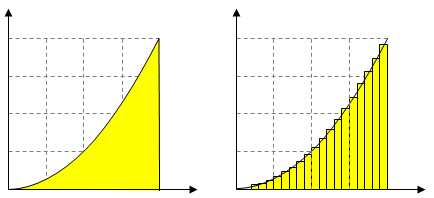# Area of Circle

## How to calculate the area of a circle

If you cut the circles and stick them together, they gradually become more rectangular. In this rectangle, the width is half of the circumference, and the height is the radius.
Therefore, The area of a circle can be calculated as shown below.

Area of ​​circle = 1/2 of circumference x radius